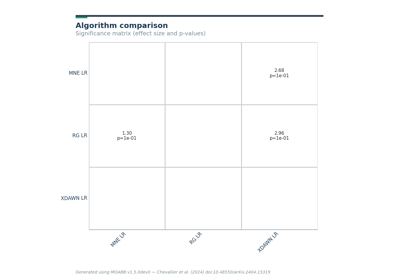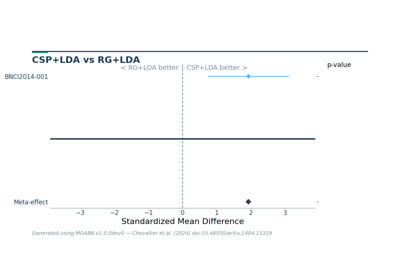# moabb.analysis.plotting.summary_plot¶

`moabb.analysis.plotting.``summary_plot`(sig_df, effect_df, p_threshold=0.05, simplify=True)[source][source]

Significance matrix to compare pipelines

Visualize significances as a heatmap with green/grey/red for significantly higher/significantly lower.

Parameters
• sig_df (DataFrame) – DataFrame of pipeline x pipeline where each value is a p-value,

• effect_df (DataFrame) – DataFrame where each value is an effect size

Returns

fig – Pyplot handle

Return type

Figure

## Examples using `moabb.analysis.plotting.summary_plot`¶